## Description

Contours are visual descriptions of glyph shapes using bezier curves.

Bezier curves were introduced into type design in the 1980s as two competing flavors, PostScript (Adobe) and TrueType (Microsoft/Apple). The PostScript format used cubic bezier curves, while the TrueType used quadratic ones. The formats evolved and were merged into the OpenType format, but the distiction remains between OpenType-CFF (cubic) and OpenType-TTF (quadratic) fonts.

The Ikarus format which existed before that was based on circle segments.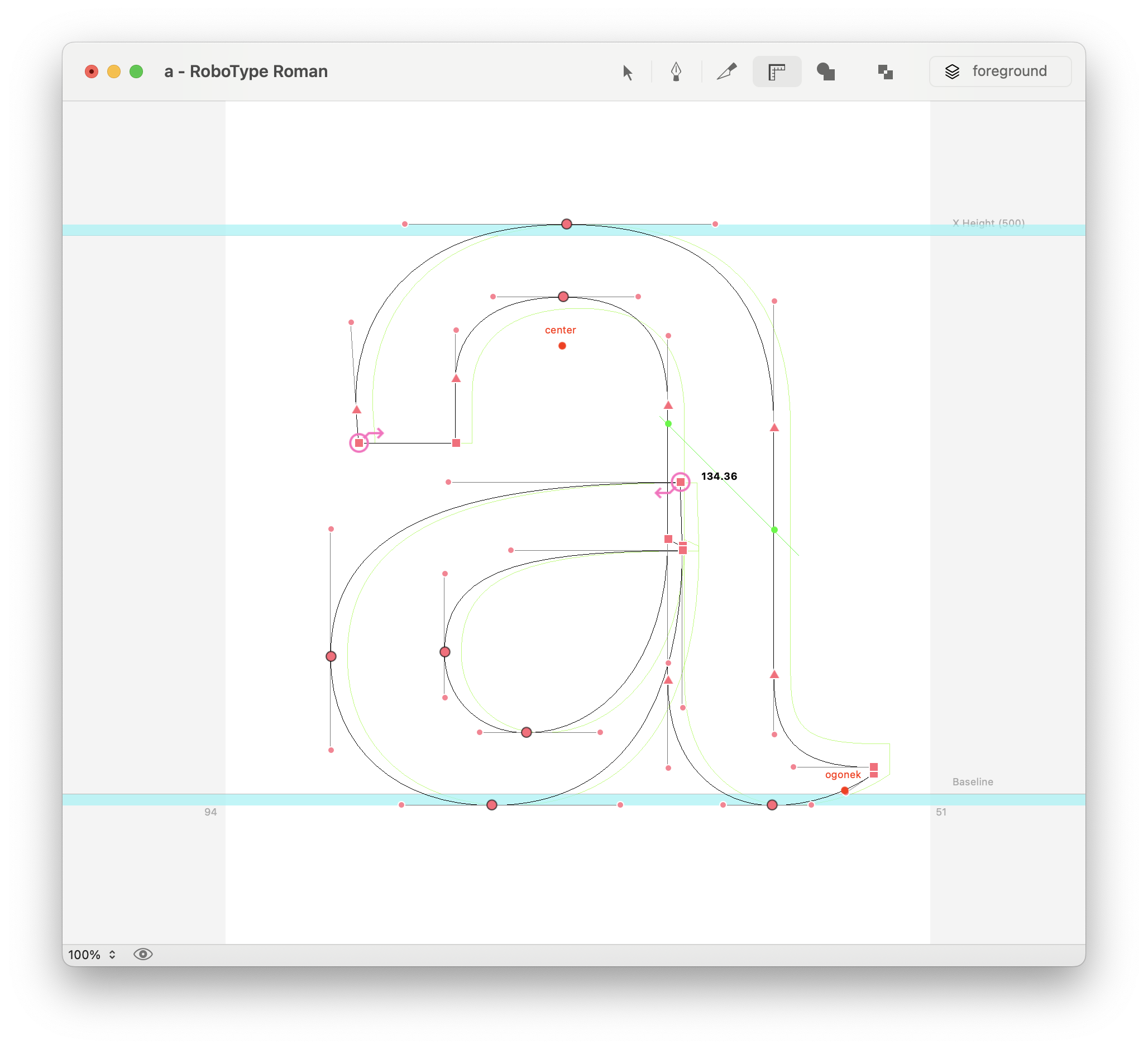Contours can be made of line and curve segments.

Line segments
are made of two on-curve points
Curve segments
are made of two on-curve points and off-curve points

Contours can be open or closed. Open contours are allowed in UFO, but not in OpenType.

RoboFont uses cubic bezier curves by default. This is the type of contours which is most familiar to designers, since it’s used by vector design applications. Cubic beziers are part of Adobe’s PostScript page description language, and are used by OpenType-CFF fonts.

Since version 4.0, RoboFont offers full support for quadratic bezier curves. This allows for a finer control over outlines meant to be exported as TrueType fonts.cubic curves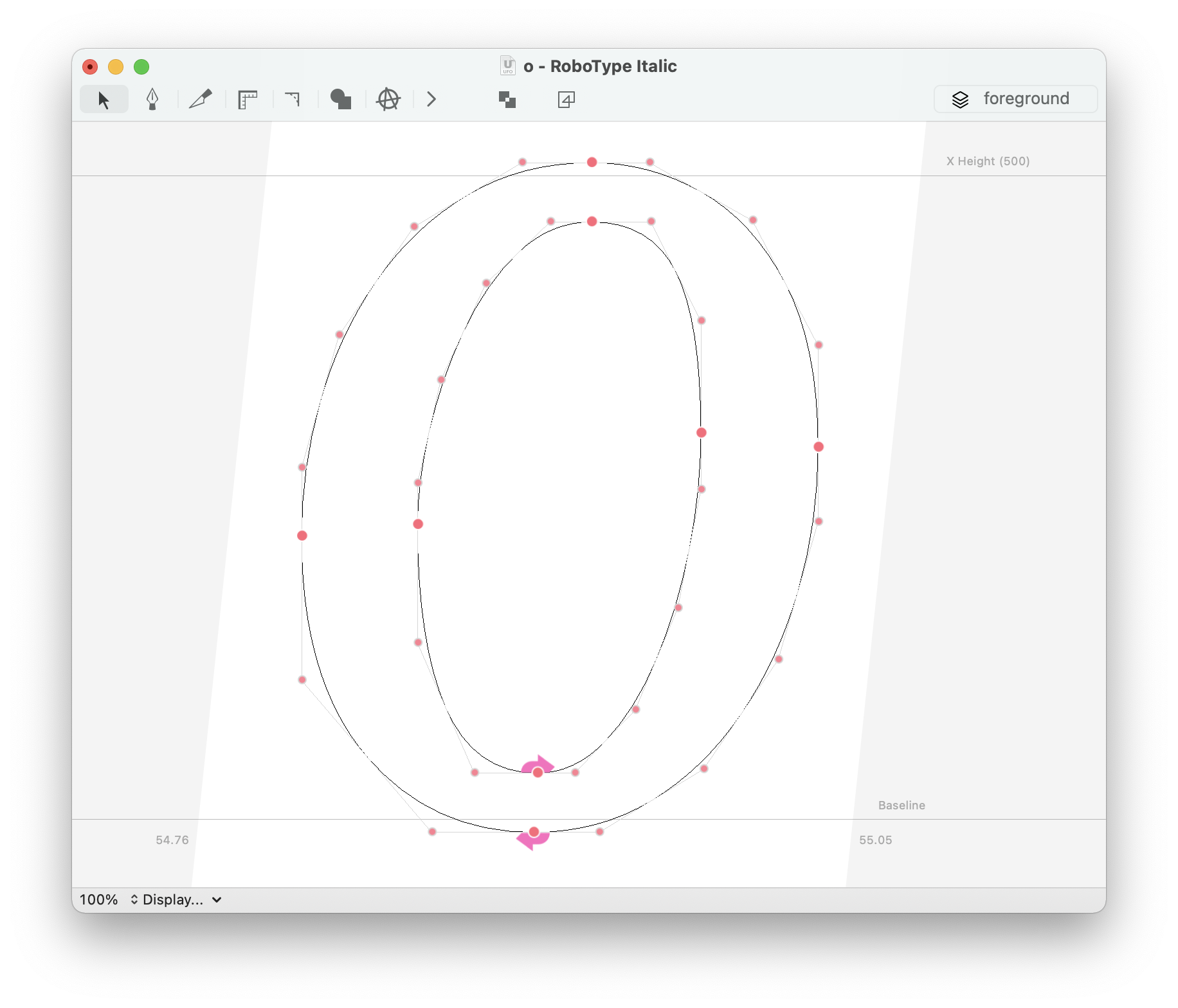• UFO3 specification allows the existence of both quadratics and beziers segments in the same contour. RoboFont abides to the specification, be aware!

### Point smoothness

Bezier points can be smooth or unsmooth.

smooth point
segments are continuous
unsmooth point
segments are at an angle

Point smoothness can be toggled on / off by double clicking the point.

Smooth points retain continuity between segments when its handles are moved.

The angle of handle is locked to the angle of the opposing line segment of or to the angle of the handle of the oppposing curve segment.

### Point representations

Points are represented by different icons depending on their smoothness and the type of segments they connect: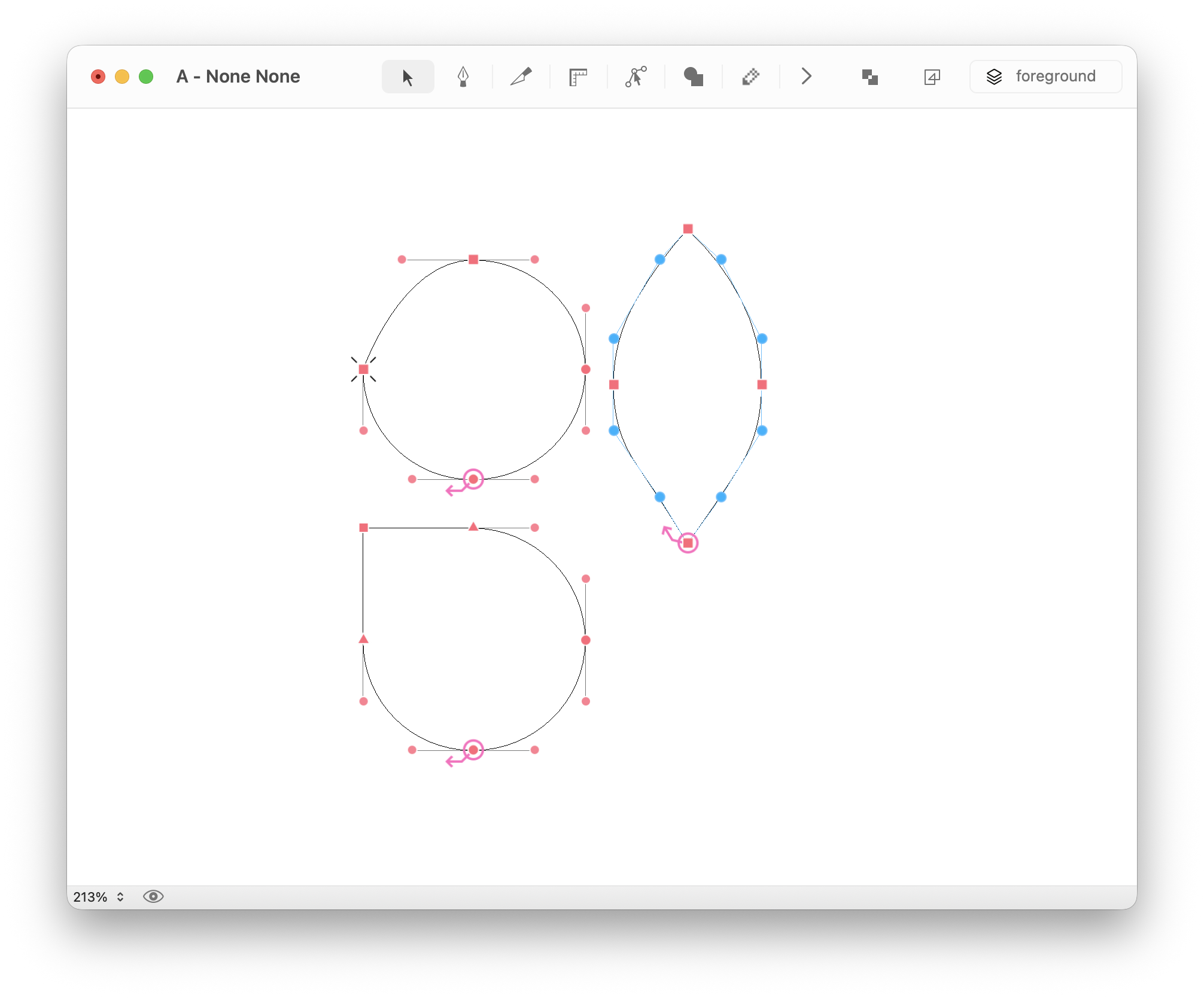Icon Description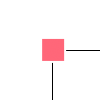Square

Unsmooth connection between line and curve segments, or two line segments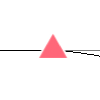Triangle

Smooth connection between line segment and curve segment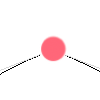Circle without stroke

Unsmooth connection between two curve segments, handles can move independently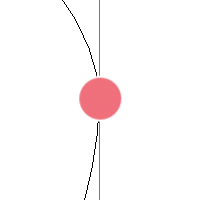Circle with stroke

Smooth connection between two curve segments, handles locked to the same angle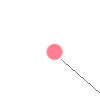Control point only connected to anchor

Cubic bezier control points. Cubic beziers only allow for two control points, each one connected to an anchor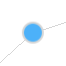Connected control points

Quadratic bezier control point. Quadratic beziers allow for an infinite amount of control points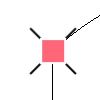Circle or square with cross underneath

On-curve point matching with off-curve pointCircle around on-curve or off-curve point

Signals the first point in the contour, either on-curve or off-curve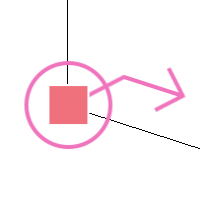Arrow

Placed on the first on-curve point of the contour (there might be off-curve points preceding it), it indicates the direction of the contour

## Creating and editing contours

Contours are usually created using the Bezier Tool and edited using the Editing Tool. It is also possible to draw inside a glyph with code – see this example.

## Contours Inspector

Contour and point properties can be edited in the Points section of the Inspector.## First Contour Point

According to the UFO specification, an open contour must start with a `move` type point:

``````<?xml version='1.0' encoding='UTF-8'?>
<glyph name="contours" format="2">
<outline>
<contour>
<point x="144" y="314" type="move"/>
<point x="204" y="159" type="line"/>
<point x="251" y="117"/>
<point x="308" y="100"/>
<point x="377" y="106" type="curve"/>
</contour>
</outline>
</glyph>
``````

while a closed contour can start with either `curve`, `line` or `qCurve` point

``````<?xml version='1.0' encoding='UTF-8'?>
<glyph name="contours" format="2">
<outline>
<contour>
<point x="120" y="167" type="line"/>
<point x="64" y="314" type="line"/>
<point x="96" y="150" type="line"/>
</contour>
<contour>
<point x="164" y="314" type="curve"/>
<point x="224" y="159" type="line"/>
<point x="178" y="203"/>
<point x="158" y="254"/>
</contour>
<contour>
<point x="324" y="159" type="qcurve"/>
<point x="264" y="314" type="line"/>
<point x="260" y="269"/>
<point x="290" y="192"/>
</contour>
</outline>
</glyph>
``````

If you want to inspect closely the content of a glif file inside a UFO, you can use the glifViewer extension by Frederik Berlaen. Using the same extension, you can paste these examples and observe the outlines directly in RoboFont

As you can see from the previous examples, off-curve points do not have a type attribute, as they can be inferred based on their relative position to the on-curve points. So, it is possible to move an off-curve point at the top of the list without breaking the UFO specification.

``````<?xml version='1.0' encoding='UTF-8'?>
<glyph name="contours" format="2">
<outline>
<contour>
<point x="260" y="269"/>
<point x="290" y="192"/>
<point x="324" y="159" type="qcurve"/>
<point x="264" y="314" type="line"/>
</contour>
</outline>
</glyph>
``````

This is not an issue while interpolating outlines with RoboFont or Skateboard, because their underlying API – fontMath – automatically picks the first on-curve point as the first contour point. Differently, tools like fontMake require strict compatibility between outlines, so if contour FOO starts with an off-curve point, contour BAR should do the same.

To provide finer control over outlines compatibility, RoboFont visualizes the first point of the contour on an off-curve point if necessary, which will detach the “first contour point” icon from the arrow that indicates the contour direction.The contextual menu can be opened with a right-click using the Editing Tool. A different set of options is displayed depending on the context: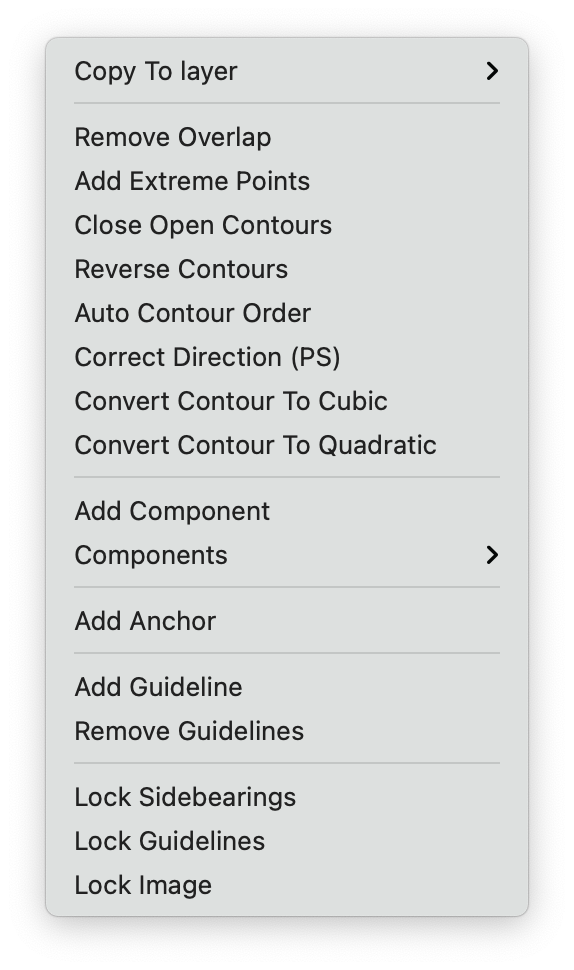no selection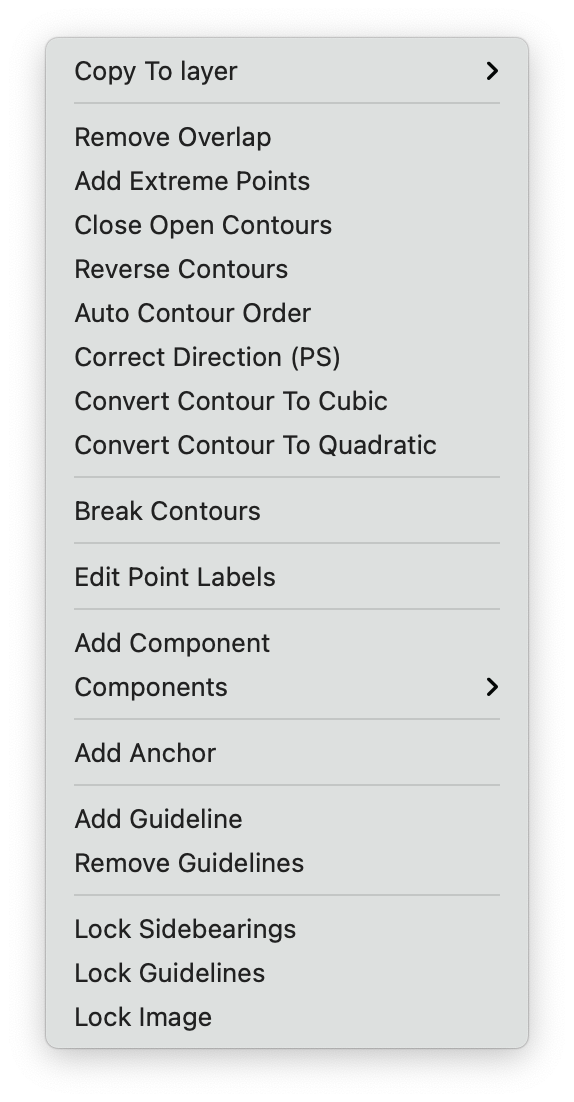contour / multiple points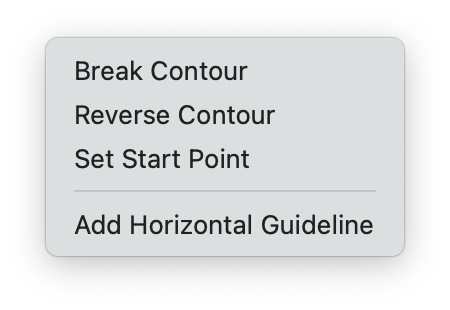single point

### Contour / Multiple points selection actions

action description
Remove Overlap Remove overlaps in the selected contours
Close Open Contours Connects two start or end points included in the selection, giving priority to start and end points of the same open contour. The selection might include non-end points, they will be ignored
Reverse Contours Reverse selected contours
Convert Contour to Cubic Convert selected contour or segments to cubic